# fitdist

## 语法

``pd = fitdist(x,distname)``
``pd = fitdist(x,distname,Name,Value)``
``[pdca,gn,gl] = fitdist(x,distname,'By',groupvar)``
``[pdca,gn,gl] = fitdist(x,distname,'By',groupvar,Name,Value)``

## 说明

``pd = fitdist(x,distname)` 通过对列向量 `x` 中的数据进行 `distname` 指定的分布拟合，创建概率分布对象。`

``pd = fitdist(x,distname,Name,Value)` 使用一个或多个名称-值对组参数指定的附加选项创建概率分布对象。例如，您可以为迭代拟合算法指示删失数据或指定控制参数。`

``[pdca,gn,gl] = fitdist(x,distname,'By',groupvar)` 基于分组变量 `groupvar` 对 `x` 中的数据进行 `distname` 指定的分布拟合，以创建概率分布对象。它返回拟合后的概率分布对象的元胞数组 `pdca`、组标签的元胞数组 `gn` 以及分组变量水平的元胞数组 `gl`。`

``[pdca,gn,gl] = fitdist(x,distname,'By',groupvar,Name,Value)` 使用一个或多个名称-值对组参数指定的附加选项返回上述输出参数。例如，您可以为迭代拟合算法指示删失数据或指定控制参数。`

## 示例

```load patients x = Weight;```

`pd = fitdist(x,'Normal')`
```pd = NormalDistribution Normal distribution mu = 154 [148.728, 159.272] sigma = 26.5714 [23.3299, 30.8674] ```

`methods(pd)`
```Methods for class prob.NormalDistribution: cdf gather icdf iqr mean median negloglik paramci pdf plot proflik random std truncate var ```

`ci95 = paramci(pd)`
```ci95 = 2×2 148.7277 23.3299 159.2723 30.8674 ```

`ci99 = paramci(pd,'Alpha',.01)`
```ci99 = 2×2 147.0213 22.4257 160.9787 32.4182 ```

```x_values = 50:1:250; y = pdf(pd,x_values); plot(x_values,y)```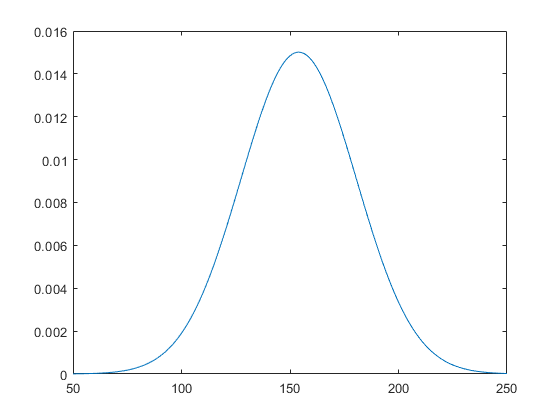`histfit(x)`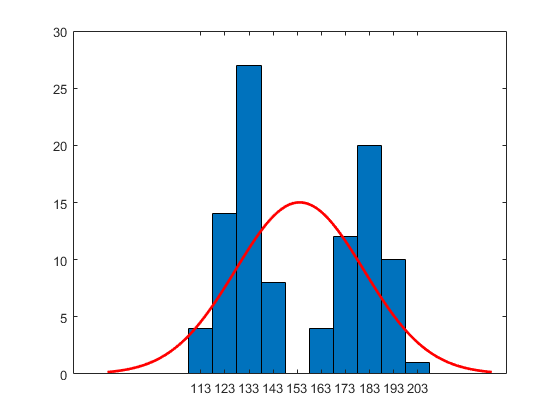`qqplot(x,pd)`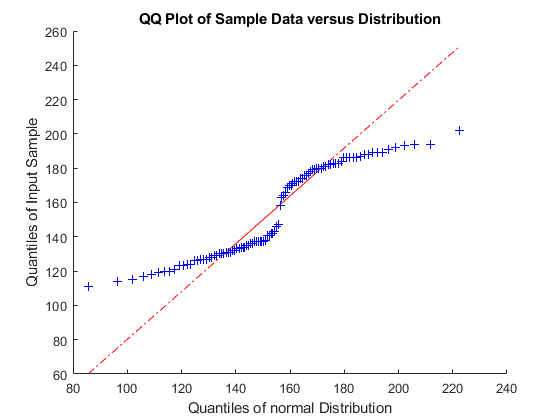```load patients x = Weight;```

`pd = fitdist(x,'Kernel','Kernel','epanechnikov')`
```pd = KernelDistribution Kernel = epanechnikov Bandwidth = 14.3792 Support = unbounded ```

```x_values = 50:1:250; y = pdf(pd,x_values); plot(x_values,y)```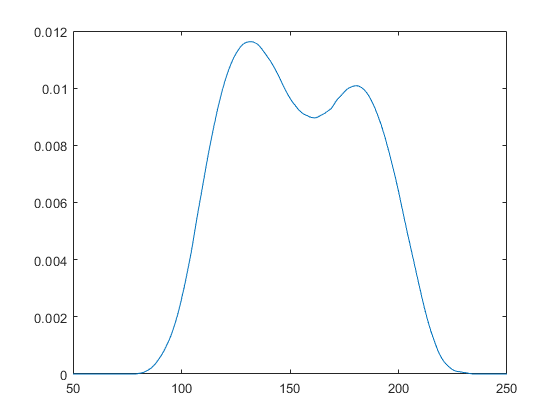```load patients x = Weight;```

`[pdca,gn,gl] = fitdist(x,'Normal','By',Gender)`
```pdca=1×2 cell array {1x1 prob.NormalDistribution} {1x1 prob.NormalDistribution} ```
```gn = 2x1 cell {'Male' } {'Female'} ```
```gl = 2x1 cell {'Male' } {'Female'} ```

`female = pdca{1} % Distribution for females`
```female = NormalDistribution Normal distribution mu = 180.532 [177.833, 183.231] sigma = 9.19322 [7.63933, 11.5466] ```
`male = pdca{2} % Distribution for males`
```male = NormalDistribution Normal distribution mu = 130.472 [128.183, 132.76] sigma = 8.30339 [6.96947, 10.2736] ```

```x_values = 50:1:250; femalepdf = pdf(female,x_values); malepdf = pdf(male,x_values);```

```figure plot(x_values,femalepdf,'LineWidth',2) hold on plot(x_values,malepdf,'Color','r','LineStyle',':','LineWidth',2) legend(gn,'Location','NorthEast') hold off```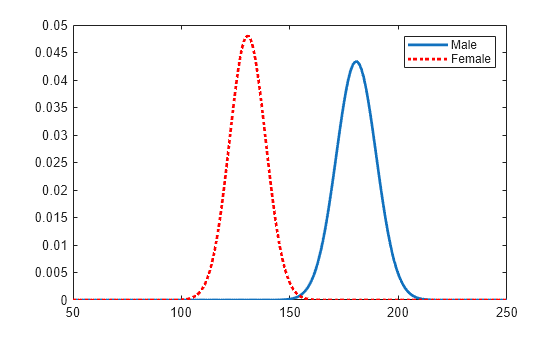```load patients x = Weight;```

`[pdca,gn,gl] = fitdist(x,'Kernel','By',Gender,'Kernel','triangle');`

`female = pdca{1} % Distribution for females`
```female = KernelDistribution Kernel = triangle Bandwidth = 5.08961 Support = unbounded ```
`male = pdca{2} % Distribution for males`
```male = KernelDistribution Kernel = triangle Bandwidth = 4.25894 Support = unbounded ```

```x_values = 50:1:250; femalepdf = pdf(female,x_values); malepdf = pdf(male,x_values);```

```figure plot(x_values,femalepdf,'LineWidth',2) hold on plot(x_values,malepdf,'Color','r','LineStyle',':','LineWidth',2) legend(gn,'Location','NorthEast') hold off```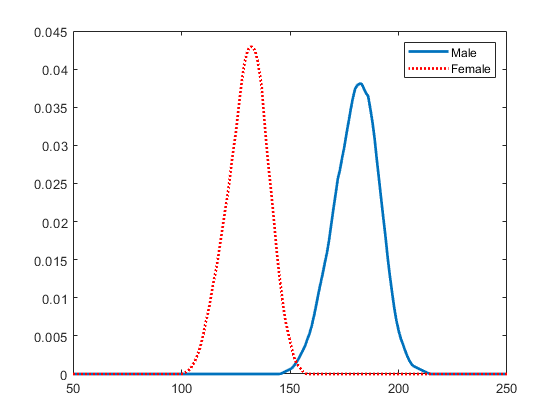## 输入参数

`'Beta'`beta 分布`BetaDistribution`
`'Binomial'`二项分布`BinomialDistribution`
`'BirnbaumSaunders'`伯恩鲍姆-桑德斯分布`BirnbaumSaundersDistribution`
`'Burr'`伯尔分布`BurrDistribution`
`'Exponential'`指数分布`ExponentialDistribution`
`'Extreme Value'``'ev'`极值分布`ExtremeValueDistribution`
`'Gamma'`gamma 分布`GammaDistribution`
`'Generalized Extreme Value'``'gev'`广义极值分布`GeneralizedExtremeValueDistribution`
`'Generalized Pareto'``'gp'`广义帕累托分布`GeneralizedParetoDistribution`
`'Half Normal'``'hn'`半正态分布`HalfNormalDistribution`
`'InverseGaussian'`逆高斯分布`InverseGaussianDistribution`
`'Kernel'`核分布`KernelDistribution`
`'Logistic'`逻辑分布`LogisticDistribution`
`'Loglogistic'`对数逻辑分布`LoglogisticDistribution`
`'Lognormal'`对数正态分布`LognormalDistribution`
`'Nakagami'`Nakagami 分布`NakagamiDistribution`
`'Negative Binomial'``'nbin'`负二项分布`NegativeBinomialDistribution`
`'Normal'`正态分布`NormalDistribution`
`'Poisson'`泊松分布`PoissonDistribution`
`'Rayleigh'`瑞利分布`RayleighDistribution`
`'Rician'`莱斯分布`RicianDistribution`
`'Stable'`稳定分布`StableDistribution`
`'tLocationScale'`t 位置尺度分布`tLocationScaleDistribution`
`'Weibull'``'wbl'`威布尔分布`WeibullDistribution`

### 名称-值参数

`fitdist` 会忽略此删失向量中的任何 `NaN` 值。此外，`x` 或频率向量中的任何 `NaN` 值都会导致 `fitdist` 忽略删失向量中的对应值。

`fitdist` 忽略此频率向量中的任何 `NaN` 值。此外，`x` 或删失向量中的任何 `NaN` 值都会导致 `fitdist` 忽略频率向量中的对应值。

• `'normal'`

• `'box'`

• `'triangle'`

• `'epanechnikov'`

`'unbounded'`密度可以取任意实数。
`'positive'`密度仅限于正值。

## 输出参数

`distname` 指定类型的概率分布对象，以元胞数组形式返回。有关 `distname` 值和对应概率分布对象的列表，请参阅 `distname`

## 算法

`fitdist` 函数使用最大似然估计来拟合大多数分布。两个例外是带有未删失数据的正态分布和对数正态分布。

• 对于未删失的正态分布，sigma 参数的估计值是方差的无偏估计值的平方根。

• 对于未删失的对数正态分布，sigma 参数的估计值是数据对数的方差的无偏估计值的平方根。

## 替代功能

• 分布拟合器打开一个图形用户界面，以便您从工作区导入数据，并以交互方式对该数据进行概率分布拟合。然后，您可以将分布作为概率分布对象保存到工作区。使用 `distributionFitter` 打开分布拟合器，或点击 Apps 选项卡上的“分布拟合器”。

• 要对左删失、双删失或区间删失数据进行分布拟合，请使用 `mle`。您可以使用 `mle` 函数找到最大似然估计值，并使用 `makedist` 函数创建一个概率分布对象。有关示例，请参阅Find MLEs for Double-Censored Data

 Johnson, N. L., S. Kotz, and N. Balakrishnan. Continuous Univariate Distributions. Vol. 1, Hoboken, NJ: Wiley-Interscience, 1993.

 Johnson, N. L., S. Kotz, and N. Balakrishnan. Continuous Univariate Distributions. Vol. 2, Hoboken, NJ: Wiley-Interscience, 1994.

 Bowman, A. W., and A. Azzalini. Applied Smoothing Techniques for Data Analysis. New York: Oxford University Press, 1997.